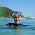## Friday, August 6, 2010

### Boltzmann and Joint Probability Distributions

One thing that struck me when reading this chapter was the comment that was made about the Boltzmann Distribution. At the bottom of page 85 it says the Boltzmann distribution is exact. Normally when I read an equation in a textbook, I assume it is quite accurate, and in an ideal world the equation I’d assume the equation is exact. But I’d also assume that if I were to make some measurements, they wouldn’t agree with the theory exactly, due mainly to my measurements, but also some small effects that the theory generalises.
Furthermore, Nelson makes this statement, but then does barely anything to justify it. It is mentioned that it can be derived from very general principles, which will be detailed in a later chapter, but that doesn’t justify why it is so exact. I can derive many things which are just approximations.

Now, I believe that the Boltzmann distribution equation is exact. I’m not disputing that fact. I just feel that that comment was a bit peculiar, as it was a strong claim made, without a lot of evidence shown to back it up. I would like to know how they can be so sure about the accuracy of this law.

On a slightly different topic: I have used probability distributions before, and when I used a discrete distribution I would use no units on my probability, and if I used a continuous one I’d use inverse measurement units, but I’d never realised that the different kinds of distributions had different units.

It wasn’t mentioned specifically, but I suspect that the probability distribution over two continuous random variables would be a two dimensional probability distribution, and have the units of inverse unit A by inverse unit B. But would the probability distribution over one continuous and one discrete random variable just have units of inverse A also? Is a joint distribution over one continuous and one discrete random variable even possible?

1.I talk about two fairly different topics in this post. Would it have been better if I made two short seperate posts? I don't want to saturate this blog with my fairly trivial thoughts.

2.Don't worry - nothing trivial here.

A good derivation of the Boltzmann distribution for the canonical ensemble can be found in the Dill & Bromberg book (supplemental text, listed in course description). There are copies in the library, my office, Alan's and Ross's. The section headings in that book are particularly clear, too.

Another good (and easy) place to look is Leonard Susskind's lectures on Statistical Mechanics in his video series entitled "Modern Physics: The Theoretical Minimum". I love these lectures. They are very clear and simple, yet not dumbed-down, and Susskind's Bronx accent is comforting (to me).

Remember that the Boltzmann distribution is just that. If you have a distribution and you want to know if it is Boltzmann's you may have to make an indefinite number of samples before the probability that it may be another distribution vanishes. However, you may realistically be able to sample enough to make yourself 99.9% confident that it is Boltzmann's distribution. The distribution itself is still EXACT, though, because it has been derived without any mathematical approximation requiring a specific limit. For example, it doesn't invoke Stirling's approximation for N!, which is only exact as N gets big.

I think you can define joint distributions for a discrete and a continuous random variable. I don't see a problem with defining the "marginal distributions" that you would get by integrating out one or the other. In this case if x is discrete and y is continuous you would get
P(y|x) continuous
P(x|y) discrete
P(x)=Integral P(x|y) dy
P(y)=Sum_x P(y|x)
without any problem I can readily see.

3.There's also an easy derivation in Eisber & Resnick (Quantum Physics of atoms, molecules, solids, nuclei and particles), which is quite nice. It approaches the topic from black body radiation. I've got a copy in my office if anyone wants to borrow it.